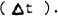Click here to make tpub.com your Home Page Page Title: Load limiter; energy absorber. Back | Up | NextWeb www.tpub.comHome

Information Categories
Aerographer
Automotive
Aviation
Construction
Diving
Draftsman
Engineering
Electronics
Food and Cooking
Logistics
Math
Medical
Music
Nuclear Fundamentals
Photography
ReligionMIL-S-8551O(AS)
a.
Establish the calibration baseline, correcting for any gravity
bias acceleration,
Establish the maximum (peak) acceleration magnitude.
b.
c.
Construct a reference line parallel to the calibration baseline
at a magnitude equal to 10 percent of the peak acceleration.  The
intersection of this line with the acceleration time plot defines
points 1 and 2.
Construct a second reference line parallel to the calibration
d.
baseline at a magnitude equal to 90 percent of the peak acceler-
ation.  The intersection of this line with the acceleration time
plot defines points 3 and 4.
e.
Construct the onset line defined by the straight line through
points 1 and 3.
Construct the offset line defined by the straight line through
f.
points 2 and 4.
Construct a line parallel to the calibration baseline, through
g.
the peak acceleration.  The time interval defined by the inter-
sections of this line with the constructed onset and offset lines
(points 5 and 6) is the plateau
h,
Locate the intersection of the constructed onset line with the
calibration baseline (point 7).  The time interval defined by
points 7 and 5 is the rise time (TR).
For a given plot of accelerations in the x and y axes, the
i.
-
specific G values are graphically obtained from the constructed
onset and offset lines for the specific time at which the sum-
mation vector "of acceleration is the greatest.
These are interchangeable names of devicesIntegrated Publishing, Inc. - A (SDVOSB) Service Disabled Veteran Owned Small Business